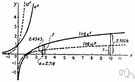# logarithm

(redirected from Logarithm of a number)
Also found in: Thesaurus, Medical, Encyclopedia.

## log·a·rithm

(lô′gə-rĭth′əm, lŏg′ə-)
n. Mathematics
The power to which a base, such as 10, must be raised to produce a given number. If nx = a, the logarithm of a, with n as the base, is x; symbolically, logn a = x. For example, 103 = 1,000; therefore, log10 1,000 = 3. The kinds most often used are the common logarithm (base 10), the natural logarithm (base e), and the binary logarithm (base 2).

[New Latin logarithmus : Greek logos, reason, proportion; see leg- in Indo-European roots + Greek arithmos, number; see ar- in Indo-European roots.]

## logarithm

(ˈlɒɡəˌrɪðəm)
n
(Mathematics) the exponent indicating the power to which a fixed number, the base, must be raised to obtain a given number or variable. It is used esp to simplify multiplication and division: if ax = M, then the logarithm of M to the base a (written logaM) is x. Often shortened to: log See also common logarithm, natural logarithm
[C17: from New Latin logarithmus, coined 1614 by John Napier, from Greek logos ratio, reckoning + arithmos number]

## log•a•rithm

(ˈlɔ gəˌrɪð əm, -ˌrɪθ-, ˈlɒg ə-)

n.
the exponent of the power to which a base number must be raised to equal a given number; log: 2 is the logarithm of 100 to the base 10 (2 = log10 100).
[1605–15; < New Latin logarithmus < Greek log- log- + arithmós number; see arithmetic]

## log·a·rithm

(lô′gə-rĭth′əm)
The power to which a base must be raised to produce a given number. For example, if the base is 10, then 3 is the logarithm of 1,000 (written log 1,000 = 3) because 103 = 1,000.

## logarithm

- From Greek logos, "reckoning, ratio," and arithmos, "number."
ThesaurusAntonymsRelated WordsSynonymsLegend:
 Noun 1logarithm - the exponent required to produce a given numberlogexponent, index, power - a mathematical notation indicating the number of times a quantity is multiplied by itselfcommon logarithm - a logarithm to the base 10Napierian logarithm, natural logarithm - a logarithm to the base e
Translations
لوغاريثْم
logaritmus
logaritme
logaritmi
logaritam
logaritmus
lógaritmi
logaritmas
logaritms
logaritmus
logaritm
ลอการิทึม

[ˈlɒgərɪθəm] N

## logarithm

[ˈlɒgərɪðəm] nlog book n
(formerly) (= registration document) [car] → carte grise
[traveller, explorer] → carnet m de route; [lorry driver] → carnet m de route
[movement of goods] → log cabin nlog fire n

nLogarithmus m

## logarithm

[ˈlɒgəˌrɪðm] nlogaritmo
common logarithm →

## logarithm

(ˈlogəriðəm) noun
(abbreviated to log (log) ) the number of times eg 10 must be multiplied by itself to produce a particular number. 10  10  10 or 103 = 1,000, so 3 is here the logarithm of 1,000.
Site: Follow: Share:
Open / Close